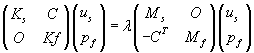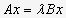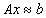# Research

### Variational Characterization for Nonlinear Eigenproblems

For a wide class of linear selfadjoint operators A on a Hilbert space the eigenvalues of the linear eigenvalue problemcan be characterized by three fundamental variational principles, namely by Rayleigh’s principle, by Poincaré’s minmax characterization, and by the maxmin principle of Courant, Fischer and Weyl.
In this project we study corresponding characterizations for nonlinear egenproblems. They are applied in vibrations of fluid–solid structures, a priori bounds for automated multi–level structuring, locking strategies for iterative projection methods, and electronic structure calculation.

### Fluid-Structure Interaction

Free vibrations of fluid-solid structures are governed by linear eigenvalue problemswhich are nonsymmetric, but are known to have real eigenvalues.
They are often tackled in the following way: one first solves the symmetric eigenproblems governing free vibrations of the fluid and of the structure independently by an efficient method like Lanczos or AMLS, and then the original problem is projected to the space spanned by the obtained eigenmodes. Hence, the coupling is not considered when constructing the search space, but only in the projected problem. In this project we study variational characterizations of the original problem, and resulting structure preserving iterative projection methods and variants of AMLS which incorporate the coupling already into the reduction process.

### Iterative Projection Methods for Nonlinear Eigenproblems

For linear sparse eigenproblemsvery efficient methods are iterative projection methods, where approximations to the wanted eigenvalues and eigenvectors are obtained from projections of the eigenproblem to subspaces of small dimension which are expanded in the course of the algorithm.
In this project we develop methods of this type for nonlinear eigenvalue problems of Arnoldi and Jacobi–Davidson type. Since no normal forms are known for this type of problems, a crucial point when approximating more than one eigenvalue is to inhibit the method to converge to the same eigenvalue repeatedly.

### Automated Multi-Level Substructuring

Over the last few years, a new method for performing frequency response and eigenvalue analysis of complex finite element (FE) structures known as Automated Multi-Level Substructuring (AMLS for short) has been developed by Bennighof an Co-workers.
In AMLS the large finite element model is recursively divided into many substructures on several levels based on the sparsity structure of the system matrices. Assuming that the interior degrees of freedom of substructures depend quasistatically on the interface degrees of freedom, and modeling the deviation from quasistatic dependence in terms of a small number of selected substructure eigenmodes, the size of the finite element model is reduced substantially yet yielding satisfactory accuracy over a wide frequency range of interest. Recent studies in vibro-acoustic analysis of passenger car bodies where very large FE models with more than one million degrees of freedom appear and several hundreds of eigenfrequencies and eigenmodes are needed have shown that AMLS is considerably faster than Lanczos type approaches for this type of problems.
Taking advantage of a variational characterization of eigenvalues for nonlinear eigenproblems we proved an a priori bound for AMLS. Our plan is to extend it to an posteriori bound and to develop efficient cut-off strategies for AMLS. Moreover, we consider generalizations of AMLS to nonlinear eigenproblemsThe efficiency of this approach was demonstrated by a gyroscopic eigenproblem modeling a rotating tire, and rational eigenproblems modeling vibrations of a fluid–solid structure and damped vibrations of a structure.

### Electron energy level calculations for semiconductor nanostructures

Semiconductor nanostructures have attracted tremendous interest in the past few years because of their special physical properties and their potential for applications in micro– and optoelectronic devices. In such nanostructures, the free carriers are confined to a small region of space by potential barriers, and if the size of this region is less than the electron wavelength, the electronic states become quantized at discrete energy levels. The ultimate limit of low dimensional structures is the quantum dot, in which the carriers are confined in all three directions, thus reducing the degrees of freedom to zero.
We consider the problem to compute relevant energy states and corresponding wave functions of (three dimensional) semiconductor nanostructures. The governing equation characterizing the energy states E and corresponding wave functionsis the Schrödinger equationwhere the confinement potential V and the electron effective mass m are discontinous across the interface between the dot and the matrix. Assuming non-parabolicity for the electron effective mass, m depends nonlinearly on the energy state E, and the Schrödinger equation becomes a rational eigenvalue problem. Discretizing by FE or FV methods it results in a nonlinear matrix eigenvalue problem which is typically large and sparse. Iterative projection methods of Arnoldi and Jacobi-Davidson type are very efficient. One of the ongoing projects is to apply the automated multi-level substructuring to this type of problems.

### Model reduction based on hierarchical substructuring

Modelling time-invariant linear systems often yields state space models of very large dimension, e.g. if they are obtained by discretizing a partial differential equation. Model reduction is an ubiquitous tool in analysis and simulation of such problems. Here the original system is approximated by one of significantly smaller order which preserves the system characteristics (e.g. stability, minimality).
In this project we study order reduction methods which are based on hierarchical substructuring as used in AMLS for huge eigenvalue problems. The original problem is recursively divided into smaller substructures on several levels, and known order reduction methods are applied to LTI-systems corresponding to these substructures. Thus, a basis of an appropriate subspace is constructed to which the original problem is projected.

### Methods for regularized total least squares problems based on eigensolvers

The total least squares method is a successful approach for linear problemsif both the system matrix A and the right hand side b are contaminated by some noise. Quite often these problems are ill-posed. Then least squares or total least squares methods often yield physically meaningless solutions, and regularization is necessary to stabilize the solution.
In this project we study and analyze methods which are based on the solution of a sequence of linear or quadratic eigenproblems. We pay particular attention to the reuse of information from previous iteration steps which may accelerate the methods considerably.